A compound A of formula C3H6Cl2 on reaction with alkali can give B of formula C3H6O or C of formula C3H4. B on oxidation gave a compound of the formula C3H6O. C with dilute H2SO4 containing Hg2+ ion gave D of formula C3H6O, which with bromine and NaOH gave the sodium salt of C2H4O2. Then A is :

(a) CH3CH2CHCl2

(b) CH3CCl2CH3

(c) CH2ClCH2CH2Cl

(d) CH3CHClCH2Cl

Concept Videos :-

#13 | Conversion: Acid Derivative to Triple Bond (n to n)

Concept Questions :-

conversion chart for n to n carbon

(a)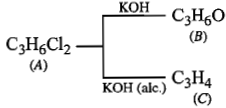or ${\mathrm{CH}}_{3}\mathrm{C}$$\equiv$CH $\underset{{\mathrm{H}}^{+},{\mathrm{Hg}}^{2+}}{\overset{{\mathrm{H}}_{2}\mathrm{O}}{\to }}$ CH3COCH$\underset{+\mathrm{NaOH}}{\overset{{\mathrm{Br}}_{2}}{\to }}$ CHBr3 +CH3COONa
Since, B and D are different thus. B is CH3CH2CHO and so A is CH3CH2CHCl2.

Difficulty Level:

• 46%
• 33%
• 15%
• 8%

Identify the product (A) in following reaction series, ${\mathrm{CH}}_{3}\mathrm{CN}\stackrel{\mathrm{Na}/{\mathrm{C}}_{2}{\mathrm{H}}_{5}\mathrm{OH}}{\to }$(X)$\stackrel{{\mathrm{HNO}}_{2}}{\to }$(Y)$\stackrel{\left[\mathrm{O}\right]}{\to }$(Z)(A) :

(a) CH3CHO

(b) CH3CONH2

(c) CH3COOH

(d) CH- CH2-NHOH

Concept Videos :-

#13 | Conversion: Acid Derivative to Triple Bond (n to n)

Concept Questions :-

conversion chart for n to n carbon

(c) ${\mathrm{CH}}_{3}\mathrm{CN}\stackrel{\mathrm{Na}/{\mathrm{C}}_{2}{\mathrm{H}}_{5}\mathrm{OH}}{\to }$${\mathrm{CH}}_{3}{\mathrm{CH}}_{2}{\mathrm{NH}}_{2}$$\stackrel{{\mathrm{HNO}}_{2}}{\to }$${\mathrm{CH}}_{3}{\mathrm{CH}}_{2}\mathrm{OH}$$\stackrel{\left[\mathrm{O}\right]}{\to }$${\mathrm{CH}}_{3}\mathrm{CHO}$${\mathrm{CH}}_{3}\mathrm{COOH}$

Difficulty Level:

• 23%
• 18%
• 50%
• 11%

In order to accomplish the following conversion, what reagent and conditions would be required?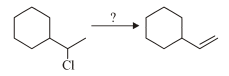1. Cold sodium hydroxide

2. Hot conc. sodium hydroxide

3. Potassium tertiary butoxide and heat

4. Hot water

Concept Videos :-

#13 | Conversion: Acid Derivative to Triple Bond (n to n)

Concept Questions :-

conversion chart for n to n carbon

(3)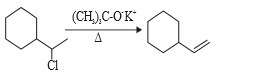Difficulty Level:

• 11%
• 36%
• 49%
• 5%

Which of the reagents shown below would accomplish the following transformations ?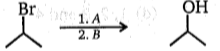A                                     B
(a) H3O+                   BH3 - THF; H2O2/NaOH
(b) NaOH                   BH3 - THF; H2O2/NaOH
(c) HBr in ether          Hg(OAc)2/H2O; NaBH4
(d) NaNH2                  Hg(OAc)2/H2O; NaBH4

Concept Videos :-

#13 | Conversion: Acid Derivative to Triple Bond (n to n)

Concept Questions :-

conversion chart for n to n carbon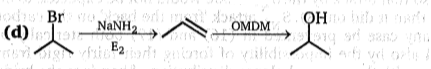Difficulty Level:

• 16%
• 34%
• 22%
• 30%

Compound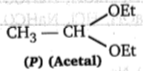and CH3-CH2-O-CH2-CH3 can be differentiated by:

(Q)

(a) H3O⊕, Na

(b) H3O⊕, Tollens' test

(c) H3O⊕, Fehling test

(d) All of these

Concept Videos :-

#13 | Conversion: Acid Derivative to Triple Bond (n to n)

Concept Questions :-

conversion chart for n to n carbon

(d)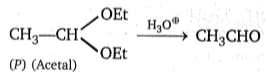; differentiated by Na, Fehling Tollen's test

CH3-CH2-O-CH2-CH3→    H3O⊕     EtOH

Difficulty Level:

• 16%
• 17%
• 9%
• 60%

Give test to differentiate 1,1-dichloroethane and 1, 2-dichloroethane:
(a) 2,4-DNP then aq. KOH
(b) aq. KOH then 2, 4-DNP
(c) NaHSO3
(d) Lucas reagent

Concept Videos :-

#13 | Conversion: Acid Derivative to Triple Bond (n to n)

Concept Questions :-

conversion chart for n to n carbon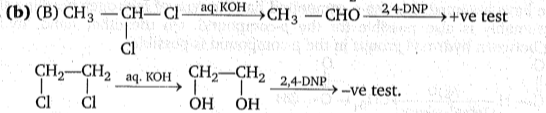Difficulty Level:

• 13%
• 68%
• 11%
• 9%

(a) sp3 to sp2

(b) sp to sp

(c) sp2 to sp

(d) sp to sp3

Concept Videos :-

#13 | Conversion: Acid Derivative to Triple Bond (n to n)

Concept Questions :-

conversion chart for n to n carbon

Difficulty Level:

• 8%
• 8%
• 79%
• 7%

The reagent used in dehydrohalogenation process is:

(a) alcoholic KOH

(b) NaNH2

(c) C2H5ONa

(d) all of these

Concept Videos :-

#13 | Conversion: Acid Derivative to Triple Bond (n to n)

Concept Questions :-

conversion chart for n to n carbon

(d) All are used as dehydrohalogenating agent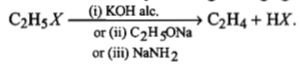Difficulty Level:

• 26%
• 7%
• 4%
• 65%

R$\to$COOH$\to$RCH2OH. This mode of reduction can be effected only by:
(a) NaBH4
(b) Na + alcohol
(c) LiAIH4
(d) all of these

Concept Videos :-

#13 | Conversion: Acid Derivative to Triple Bond (n to n)

Concept Questions :-

conversion chart for n to n carbon

(d) Na/C2H5OH, LiAlH4 or NaBH4 are used for this purpose.

Difficulty Level:

• 5%
• 6%
• 28%
• 62%

Consider the following reaction,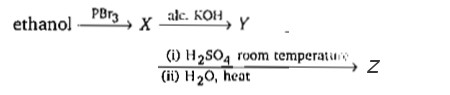the product Z, is

(1) CH2=CH2

(2) CH3CH2-O-CH2-CH3

(3) CH3-CH2-O-SO3H

(4) CH3CH2OH

Concept Videos :-

#13 | Conversion: Acid Derivative to Triple Bond (n to n)

Concept Questions :-

conversion chart for n to n carbon

KEY IDEA:

(i) PBr3 is a halogenating agent i.e., converts -OH group into-Br

(ii) Alc. KOH is a dehydrohalogenating agent.

(iii) H2SO4 and H2O converts an olefin into alcohol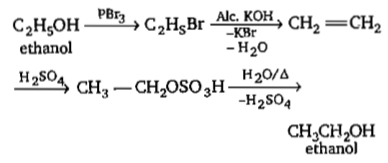Difficulty Level:

• 14%
• 15%
• 21%
• 52%